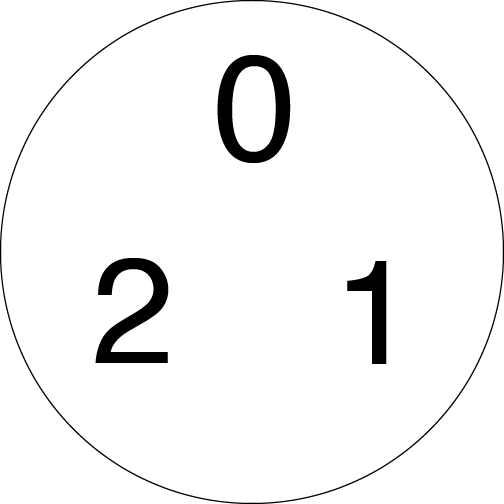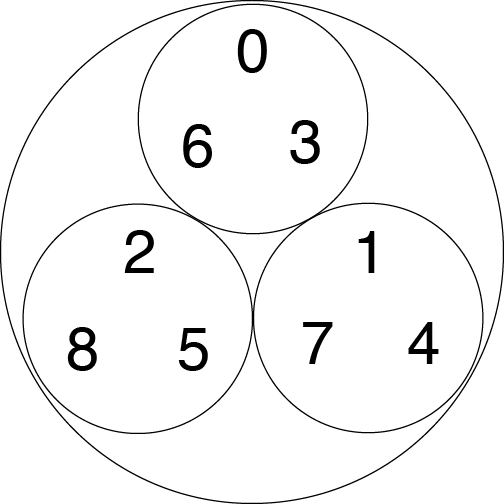Peter Scholze, one of the four Fields Medalists recognized at the International Congress of Mathematicians at the beginning of August, studies algebraic geometry. One of the motivating questions in that field is when there are whole number or rational number solutions to polynomial equations. For example, the equation x^2+y^2+z^2=1, which defines a sphere in three-dimensional space, has the point (3/13,4/13, 12/13) as a solution.

In his Fields Medal lecture, Scholze talked about his work in p-adic geometry. (You can read a survey article he wrote to accompany the lecture here, though frankly if you can read that, you don’t need to be reading my post about it because you’re well ahead of me in your understanding of the p-adics.) The p-adics are an alternative number system, sometimes more useful than the real numbers for tackling problems in algebraic geometry and number theory.

There are several roads that lead to the p-adics. One way to get there is to start with absolute values. We’re used to the standard absolute value, which measures a number’s distance from 0. If a number is greater than 0, its absolute value is just itself, and if a number is less than 0, its absolute value is the opposite of itself. So |1|=1 and |-1|=1.

We can introduce a different way of measuring a number’s size, though, based on its relationship to a number p. If you’ve taken some advanced math classes, p is probably making your spidey sense tingle. Mathematicians usually use the letter p when they’re talking about prime numbers, and for the rest of the post, we’ll just assume p is prime. When talking about the p-adics for a specific p, mathematicians replace p with the number itself, so we talk about 2-adics, 3-adics, 29-adics, 314159-adics, you get the idea. (Yeah, 314159 is prime. Even my Pi Day curmudgeon heart flutters a little at that.)

A number’s p-adic absolute value is the reciprocal of the largest power of p that divides it. This is a case where a specific example can make that complicated idea a little easier to parse. Let’s look at the 3-adics first. The number 4 isn’t divisible by any positive power of 3. It’s equal to 30x4. That means 1 is the largest power of p that divides 4, making the p-adic absolute value of 4 equal to 1/1, or 1. The number 6 is a little more interesting. It’s equal to 31x2, making its absolute value equal to 1/3. The 3-adic absolute value of 54 is 1/27 because 27 is the largest power of 3 that divides 54. Multiples of very large powers of 3 have very small p-adic absolute values.

3Blue1Brown also explains the idea of p-adic numbers, using the example of 2-adics, in this video.

The p-adic absolute value has some interesting properties the normal one doesn’t. For example, there are a lot of numbers that share an absolute value. The numbers 1, 2, 4, 5, 7, 8, and an infinite string of other numbers all have 3-adic absolute value 1. The numbers 3, 6, 12, 15, 21, 24, and so on all have absolute value 1/3. Our usual absolute value only allows us to have at most two numbers with the same absolute value. Additionally, rather than numbers being able to have a continuous range of absolute values, they have a discrete set of options. There are an infinite number of them, but any number’s size must be a power of p. So there’s no number with 3-adic absolute value of 1/2 or 7. We’re thinking about numbers in a strange and different way.

The p-adic absolute value gives us a new way to measure the distance between two numbers. The p-adic distance between two numbers x and y is the p-adic absolute value of the number x-y. So going back to the 3-adics, that means numbers are closer to each other if they differ by a large power of 3. The numbers 1 and 4 have a difference of 3, so their 3-adic distance from each other is 1/3. But the numbers 1 and 19 have a 3-adic distance of 1/9 (18=32x2), and 1 and 82 have a 3-adic distance of 1/81. The numbers 11 and 29 have a difference of 18, so they have a 3-adic distance of 1/9.

If you’ve worked with modular arithmetic, this might be feeling sort of familiar. In arithmetic modulo, or mod, the number n, you only care about how close a number is to a multiple of n. The numbers 2, 9, and 93 are equivalent mod 7 because they’re all 2 more than a multiple of 7. In some ways, the p-adic distance sort of feels like you care about numbers mod pk for all powers pk at once.

One way to try to understand a new way of measuring distance is to try to figure out what is close to what in this distance. In the 3-adic metric, positive whole numbers are a maximum of 1 unit away from each other, so we can think about all of them as living in this bounded circle.The numbers 0, 1, and 2 sitting in a 3-adic bubble. All other positive whole numbers also sit in this circle, but we can see a structure emerge in a neat way if we just start with these numbers. Credit: Evelyn Lamb

Let’s think of this circle as meaning anything within the circle is distance at most 1 away from anything else. Right now I just have the numbers 1, 2, and 3 in there, but all whole numbers 0 and larger are in there because they’re all at most distance 1 away from each other.

Now we can add another layer. The number 3 is only 1/3 away from the number 0, the number 4 is 1/3 away from 1, and 5 is 1/3 away from 2. We can add those numbers to smaller circles. If two numbers are in the same smaller circle, they have distance at most 1/3 from each other.The numbers 0 through 8 arranged 3-adically. The numbers 0, 3, and 6 (for example) are all distance 1/3 from each other, so they are in the same small circle. Credit: Evelyn Lamb

I’ll draw one more layer of this process, but we could keep going forever, yielding a lovely fractal pattern reminiscent of the Sierpinski triangle. The distance between two numbers is the size of the smallest circle that contains both of them.

Whew! That’s a lot of work, and we haven’t even gotten past positive whole numbers. But the p-adics don’t stop there! You can extend the p-adic absolute value and distance functions to all rational numbers.